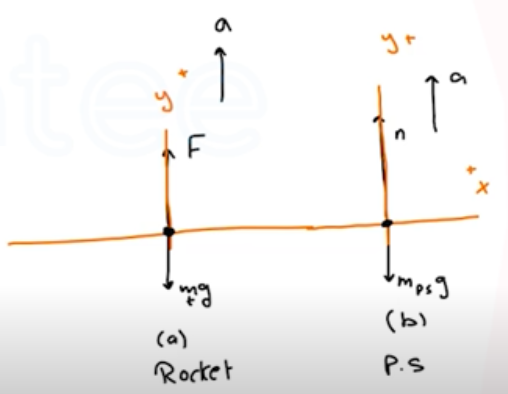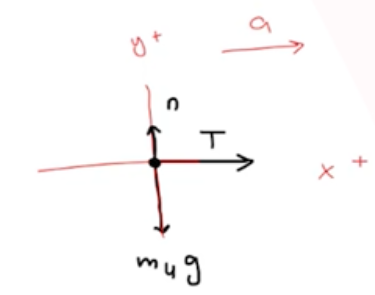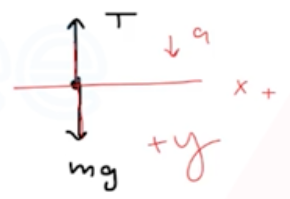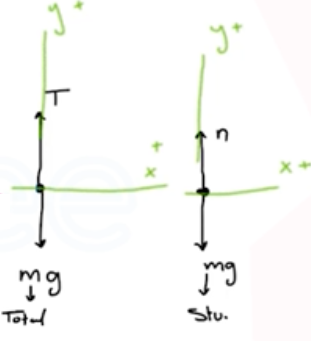Need Help?

Subscribe to Physics 1

###### \${selected_topic_name}
• Notes

$\begin{array}{l}{\text { A } 125-\mathrm{kg} \text { (including all the contents) rocket has an engine }} \\ {\text { that produces a constant vertical force (the thrust) of } 1720 \mathrm{N} \text { . }} \\ {\text { Inside this rocket, a } 15.5-\mathrm{N} \text { electrical power supply rests on the }} \\ {\text { floor. (a) Find the acceleration of the rocket. (b) When it has }} \\ {\text { reached an altitude of } 120 \mathrm{m}, \text { how hard does the floor push on the }} \\ {\text { power supply? (Hint: Start with a free-body diagram for the power }} \\ {\text { power supply? (Hint: Start with a free-body diagram for the power }} \\ {\text { supply.) }}\end{array}$$\sum F_ y= m a_ y$

$\rightarrow F-m_{t} g=m_{t} a$

$a=\frac{F-m_t g}{m_t}=\frac{1720-(125)(9.8)}{125}$

$∴ a=3.96$

$\sum F_{y}=m a_ y$

$n-m_{ p.s} g=m_{ p.s}{a}$

$n=m_{p.s} a+m_{p .s}g=m_{p.s}(a+a)$

$=(1.58)(3.96+9.8)$

$∴ n=21.7 N$

$\begin{array}{l}{\text { A light rope is attached to a block with mass } 4.00 \text { kg that }} \\ {\text { rests on a frictionless, horizontal surface. The horizontal rope }} \\ {\text { passes over a frictionless, massless pulley, and a block with mass } m} \\ {\text { is suspended from the other end. When the blocks are released, the }}\end{array}$

$\begin{array}{l}{\text { tension in the rope is } 10.0 \mathrm{N} \text { . (a) Draw two free-body diagrams, }} \\ {\text { one for the } 4.00-\mathrm{kg} \text { block and one for the block with mass } m \text { . }} \\ {\text { (b) What is the acceleration of either block? (c) Find the mass } m \text { of }} \\ {\text { the hanging block. (d) How does the tension compare to the weight }} \\ {\text { of the hanging block? }}\end{array}$$\sum \vec{F}=m \vec{a}$

1) $\sum F_{x}=m a_ x$

$T=(4) a_ x \rightarrow a_ x=\frac{T}{4}$

$∴ a_{x}=\frac{10}{4}=2.5 \mathrm{m/s}^{2}$

2) $\sum F_{y}=m a _y$

$m g-T=ma$

$T=m g-m a \rightarrow T=m(g-a)$

$m=\frac{T}{(g-a)}=\frac{10}{9.8-2.5}=1.37 k g$

$w=m g=(1.37)(9.8)=13.4 N$

$w=13.4 \mathrm{N}, \quad T=10 \mathrm{N}$

$T=0.75 w \rightarrow \frac{T}{w}=\frac{10}{13.4} \approx 0.75$

$T=0.75 w$

$\begin{array}{l}{\text { A } 550 \text { -N physics student stands on a }} \\ {\text { bathroom scale in an } 850-\mathrm{kg} \text { (including the student) elevator that is }} \\ {\text { supported by a cable. As the elevator starts moving, the scale reads }}\end{array}$$\sum F_{y}=ma_y$

$n-(m g)=m a$

$w=m g$

$550=m(9.8)$

$\rightarrow m=\frac{550}{9.8}=56.1 \mathrm{kg}$

$450-550=56.1(a)$

$a=\frac{450- 550}{56.1}=-1.78 \mathrm{m/s}^{2}$

$a=\frac{670-550}{56.1}=2.14 \mathrm{m} / \mathrm{s}^{2}$

$a=\frac{0-550}{56.1}=-9.8 \mathrm{m/s}^{2}$

$\frac{-w}{m}=a=-g$

$w=m g \rightarrow g=\frac{w}{m}$

$\sum F_ y=m a_ y \Rightarrow T-m_{total} g=m_{total}a$

$\rightarrow T=m_{t} a+m_{t}g=m_{total} (a+g)$

$=850(-1.78+9.8)=6820 \mathrm{N}$

$T=850(-9.8+9.8)=Zero$